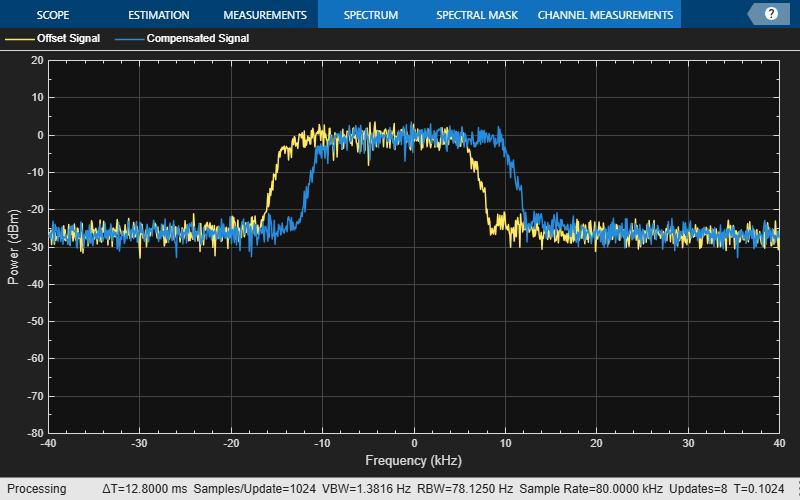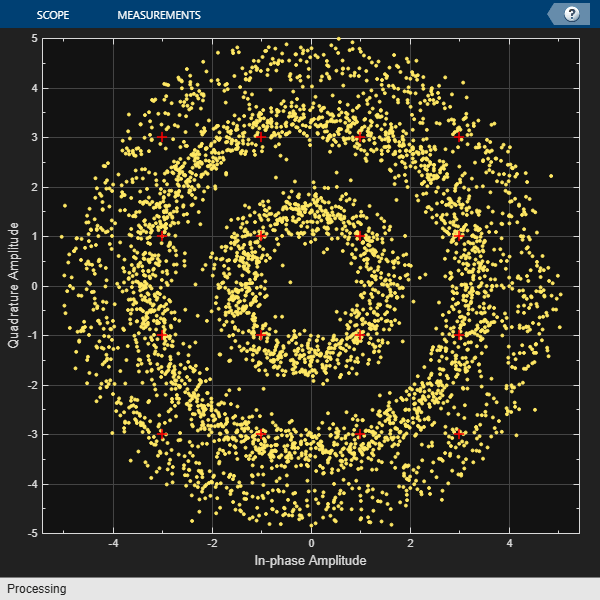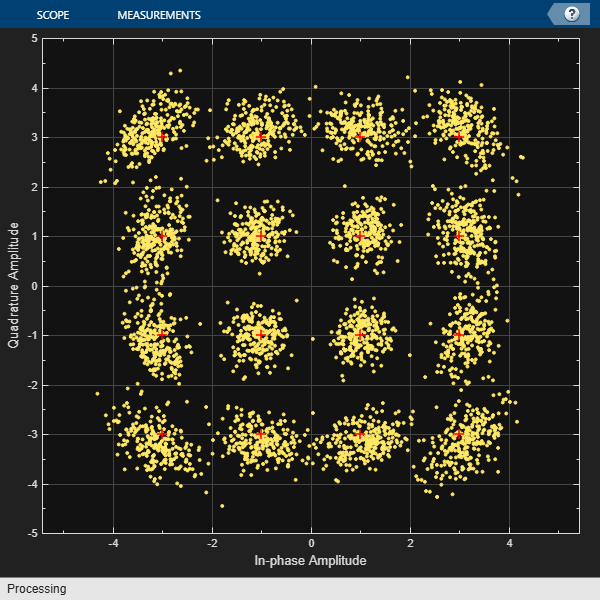Documentation

# comm.CoarseFrequencyCompensator

Compensate for frequency offset for PAM, PSK, or QAM

## Description

The `CoarseFrequencyCompensator` System object™ compensates for the frequency offset of received signals.

To compensate for the frequency offset of a PAM, PSK, or QAM signal:

1. Define and set up your coarse frequency compensator object. See Construction.

2. Call `step` to compensate for the frequency offset of a PAM, PSK, or QAM signal according to the properties of `comm.CoarseFrequencyCompensator`. The behavior of `step` is specific to each object in the toolbox.

### Note

Starting in R2016b, instead of using the `step` method to perform the operation defined by the System object, you can call the object with arguments, as if it were a function. For example, ```y = step(obj,x)``` and `y = obj(x)` perform equivalent operations.

## Construction

`CFC = comm.CoarseFrequencyCompensator` creates a coarse frequency offset compensator object, `CFC`. This object uses an open-loop technique to estimate and compensate for the carrier frequency offset in a received signal.

`CFC = comm.CoarseFrequencyCompensator(Name,Value)` creates a coarse frequency offset compensator object, `CFC`, with the specified property `Name` set to the specified `Value`. You can specify additional name-value pair arguments in any order as `Name1,Value1,...,NameN,ValueN`.

## Properties

`Modulation`

Modulation type

Specify the signal modulation type as `BPSK`, `QPSK`, `OQPSK`, `8PSK`, `PAM`, or `QAM`. The default is `QAM`. This property is nontunable.

`Algorithm`

Algorithm used to estimate frequency offset

Specify the estimation algorithm as one of `FFT-based` or `Correlation-based`. The default is `FFT-based`. This property is nontunable.

The table shows the allowable combinations of the modulation type and the estimation algorithm.

ModulationFFT-Based AlgorithmCorrelation-Based Algorithm
`BPSK`, `QPSK`, `8PSK`, `PAM`
`OQPSK`, `QAM`

Use the correlation-based algorithm for HDL implementations and for other situations in which you want to avoid using an FFT.

This property appears when `Modulation` is `'BPSK'`, `'QPSK'`, `'8PSK'`, or `'PAM'`.

`FrequencyResolution`

Frequency resolution (Hz)

Specify the frequency resolution for the offset frequency estimation as a positive, real scalar of data type `double`. This property establishes the FFT length used to perform spectral analysis and must be less than the sample rate. The default is `0.001`. This property is nontunable.

`MaximumFrequencyOffset`

Maximum measurable frequency offset (Hz)

Specify the maximum measurable frequency offset as a positive, real scalar of data type `double`.

The value of this property must be less than fsamp / M, where fsamp is the sample rate and M is the modulation order. As a best practice, set `MaximumOffset` to less than r / (4M). This property applies only if `Algorithm` is `Correlation-based`. The default is `0.05`. This property is nontunable.

`SampleRate`

Sample rate (Hz)

Specify the sample rate in samples per second as a positive, real scalar of data type `double`. The default is `1`. This property is nontunable.

`SamplesPerSymbol`

Samples per symbol

Specify the number of samples per symbol, s, as a real positive finite integer scalar, such that s ≥ 2. The default value is `4`. This property is nontunable.

This property appears when `Modulation` is `'OQPSK'`.

## Methods

 info Characteristic information about coarse frequency compensator reset Reset states of the `CoarseFrequencyCompensator` object step Compensate for frequency offset
Common to All System Objects
`release`

Allow System object property value changes

## Examples

expand all

Compensate for a 4 kHz frequency offset imposed on a noisy QPSK signal.

Set the example parameters.

```nSym = 2048; % Number of input symbols sps = 4; % Samples per symbol nSamp = nSym*sps; % Number of samples fs = 80000; % Sampling frequency (Hz) ```

Create a square root raised cosine transmit filter.

```txfilter = comm.RaisedCosineTransmitFilter(... 'RolloffFactor',0.2, ... 'FilterSpanInSymbols',8, ... 'OutputSamplesPerSymbol',sps); ```

Create a phase frequency offset object to introduce the 4 kHz frequency offset.

```freqOffset = comm.PhaseFrequencyOffset(... 'FrequencyOffset',-4000, ... 'SampleRate',fs); ```

Create a coarse frequency compensator object to compensate for the offset.

```freqComp = comm.CoarseFrequencyCompensator(... 'Modulation','QPSK', ... 'SampleRate',fs, ... 'FrequencyResolution',1); ```

Generate QPSK symbols, filter the modulated data, pass the signal through an AWGN channel, and apply the frequency offset.

```data = randi([0 3],nSym,1); modData = pskmod(data,4,pi/4); txSig = txfilter(modData); rxSig = awgn(txSig,20,'measured'); offsetData = freqOffset(rxSig); ```

Compensate for the frequency offset using `freqComp`. When the frequency offset is high, it is beneficial to do coarse frequency compensation prior to receive filtering because filtering suppresses energy in the useful spectrum.

```[compensatedData,estFreqOffset] = freqComp(offsetData); ```

Display the estimate of the frequency offset.

```estFreqOffset ```
```estFreqOffset = -3.9999e+03 ```

Return information about the `freqComp` object. To obtain the FFT length, you must call `freqComp` prior to calling the `info` method.

```freqCompInfo = info(freqComp) ```
```freqCompInfo = struct with fields: FFTLength: 131072 Algorithm: 'FFT-based' ```

Create a spectrum analyzer object and plot the offset and compensated spectra. Verify that the compensated signal has a center frequency at 0 Hz and that the offset signal has a center frequency at -4 kHz.

```specAnal = dsp.SpectrumAnalyzer('SampleRate',fs,'ShowLegend',true, ... 'ChannelNames',{'Offset Signal' 'Compensated Signal'}); specAnal([offsetData compensatedData]) ```Correct for a phase and frequency offset in a noisy QAM signal using a carrier synchronizer. Then correct for the offsets using both a carrier synchronizer and a coarse frequency compensator.

Set the example parameters.

```fs = 10000; % Symbol rate (Hz) sps = 4; % Samples per symbol M = 16; % Modulation order k = log2(M); % Bits per symbol ```

Create a QAM modulator and an AWGN channel.

```channel = comm.AWGNChannel('EbNo',20,'BitsPerSymbol',k,'SamplesPerSymbol',sps); ```

Create a constellation diagram object to visualize the effects of the offset compensation techniques. Specify the constellation diagram to display only the last 4000 samples.

```constdiagram = comm.ConstellationDiagram(... 'ReferenceConstellation',qammod(0:M-1,M), ... 'SamplesPerSymbol',sps, ... 'SymbolsToDisplaySource','Property','SymbolsToDisplay',4000, ... 'XLimits',[-5 5],'YLimits',[-5 5]); ```

Introduce a frequency offset of 400 Hz and a phase offset of 30 degrees.

```phaseFreqOffset = comm.PhaseFrequencyOffset(... 'FrequencyOffset',400,... 'PhaseOffset',30,... 'SampleRate',fs); ```

Generate random data symbols and apply 16-QAM modulation.

```data = randi([0 M-1],10000,1); modSig = qammod(data,M); ```

Create a raised cosine filter object and filter the modulated signal.

```txfilter = comm.RaisedCosineTransmitFilter('OutputSamplesPerSymbol',sps, ... 'Gain',sqrt(sps)); txSig = txfilter(modSig); ```

Apply the phase and frequency offset, and then pass the signal through the AWGN channel.

```freqOffsetSig = phaseFreqOffset(txSig); rxSig = channel(freqOffsetSig); ```

Apply fine frequency correction to the signal by using the carrier synchronizer.

```fineSync = comm.CarrierSynchronizer('DampingFactor',0.7, ... 'NormalizedLoopBandwidth',0.005, ... 'SamplesPerSymbol',sps, ... 'Modulation','QAM'); rxData = fineSync(rxSig); ```

Display the constellation diagram of the last 4000 symbols.

```constdiagram(rxData) ```Even with time to converge, the spiral nature of the plot shows that the carrier synchronizer has not yet compensated for the large frequency offset. The 400 Hz offset is 1% of the sample rate.

Repeat the process with a coarse frequency compensator inserted before the carrier synchronizer.

Create a coarse frequency compensator to reduce the frequency offset to a manageable level.

```coarseSync = comm.CoarseFrequencyCompensator('Modulation','QAM','FrequencyResolution',1,'SampleRate',fs*sps); ```

Pass the received signal to the coarse frequency compensator and then to the carrier synchronizer.

```syncCoarse = coarseSync(rxSig); rxData = fineSync(syncCoarse); ```

Plot the constellation diagram of the signal after coarse and fine frequency compensation.

```constdiagram(rxData) ```The received data now aligns with the reference constellation.

## Algorithms

### Correlation-Based

The correlation-based estimation algorithm, which can be used to estimate the frequency offset for PSK and PAM signals, is described in . To determine the frequency offset, Δf, the algorithm performs a maximum likelihood (ML) estimation of the complex-valued oscillation `exp`(j2πΔft). The observed signal, rk, is represented as

`${r}_{k}={e}^{j\left(2\pi \Delta fk{\text{T}}_{s}+\theta \right)},\text{\hspace{0.17em}}1\le k\le N\text{\hspace{0.17em}},$`

where Ts is the sampling interval, θ is an unknown random phase, and N is the number of samples. The maximum likelihood estimation of the frequency offset is equivalent to seeking the maximum of the likelihood function, Λ(Δf),

`$\Lambda \left(\Delta f\right)\approx {|\sum _{i=1}^{N}{r}_{i}{e}^{-j2\pi \Delta fi{T}_{s}}|}^{2}=\sum _{k=1}^{N}\sum _{m=1}^{N}{r}_{k}{r}_{m}^{*}{e}^{-j2\pi \Delta f{T}_{s}\left(k-m\right)}\text{\hspace{0.17em}}.$`

After simplifying, the problem is expressed as a discrete Fourier transform, weighted by a parabolic windowing function. It is expressed as

`$\mathrm{Im}\left\{\sum _{k=1}^{N-1}k\left(N-k\right)R\left(k\right){e}^{j2\pi \Delta \stackrel{^}{f}{T}_{s}}\right\}=0\text{\hspace{0.17em}},$`

where R(k) denotes the estimated autocorrelation of the sequence rk and is represented as

`$R\left(k\right)\triangleq \frac{1}{N-k}\sum _{i=k+1}^{N}{r}_{i}\text{\hspace{0.17em}}{r}_{i-k}^{*},\text{\hspace{0.17em}}0\le k\le N-1\text{\hspace{0.17em}}.$`

The term k(N–k) is the parabolic windowing function. In , it is shown that R(k) is a poor estimate of the autocorrelation of rk when k = 0 or when k is close to N. Consequently, the windowing function can be expressed as a rectangular sequence of 1s for k = 1, 2, ..., L, where LN – 1. The results is a modified ML estimation strategy in which

`$\mathrm{Im}\left\{\sum _{k=1}^{L}R\left(k\right){e}^{-j2\pi \Delta \stackrel{^}{f}k{T}_{s}}\right\}=0\text{\hspace{0.17em}}.$`

This results in an estimate of $\Delta \stackrel{^}{f}$ in which

`$\Delta \stackrel{^}{f}\cong \frac{{f}_{samp}}{\pi \left(L+1\right)}\mathrm{arg}\left\{\sum _{k=1}^{L}R\left(k\right)\right\}\text{\hspace{0.17em}}.$`

The sampling frequency, fsamp, is the reciprocal of Ts. The number of elements used to compute the autocorrelation sequence, L, are determined as

`$L=\mathrm{round}\left(\frac{{f}_{samp}}{{f}_{max}}\right)-1,\text{\hspace{0.17em}}$`

where fmax is the maximum expected frequency offset and `round` is the nearest integer function. The frequency offset estimate improves when L ≥ 7 and leads to the recommendation that fmaxfsamp / (4M).

### FFT-Based

FFT-based algorithms can be used to estimate the frequency offset for all modulation types. Two variations are used in comm.CoarseFrequencyCompensator.

• For `BPSK`, `QPSK`, `8PSK`, `PAM`, or `QAM` modulations the FFT-based algorithm used is described in . The algorithm estimates $\Delta \stackrel{^}{f}$ by using a periodogram of the mth power of the received signal and is given as

`$\Delta \stackrel{^}{f}=\frac{{f}_{samp}}{N\cdot m}\mathrm{arg}\underset{f}{\mathrm{max}}|\sum _{k=0}^{N-1}{r}^{m}\left(k\right){e}^{-j2\pi kt/N}|,\text{ }\left(-\frac{{R}_{sym}}{2}\le f\le \frac{{R}_{sym}}{2}\right)\text{\hspace{0.17em}},$`

where m is the modulation order, r(k) is the received sequence, Rsym is the symbol rate, and N is the number of samples. The algorithm searches for a frequency that maximizes the time average of the mth power of the received signal multiplied by various frequencies in the range of [–Rsym/2, Rsym/2]. As the form of the algorithm is the definition of the discrete Fourier transform of rm(t), searching for a frequency that maximizes the time average is equivalent to searching for a peak line in the spectrum of rm(t). The number of points required by the FFT is

`$N={2}^{⌈{\mathrm{log}}_{2}\left(\frac{{f}_{samp}}{{f}_{r}}\right)⌉}\text{\hspace{0.17em}},$`

where fr is the desired frequency resolution.

• For `OQPSK` modulation the FFT-based algorithm used is described in . The algorithm searches for spectral peaks at +/- 200 kHz around the symbol rate. This technique locates desired peaks in the presence of interference from spectral content around baseband frequencies due to filtering.

## References

 Luise, M. and R. Regiannini. “Carrier recovery in all-digital modems for burst-mode transmissions.” IEEE® Transactions on Communications. Vol. 43, No. 2, 3, 4, Feb/Mar/April, 1995, pp. 1169–1178.

 Wang, Y., K. Shi, and E. Serpedi. “Non-Data-Aided Feedforward Carrier Frequency Offset Estimators for QAM Constellations: A Nonlinear Least-Squares Approach.” EURASIP Journal on Applied Signal Processing. 2004:13, pp. 1993–2001.

 Nakagawa, T., M. Matsui, T. Kobayashi, K. Ishihara, R. Kudo, M. Mizoguchi, and Y. Miyamoto. “Non-Data-Aided Wide-Range Frequency Offset Estimator for QAM Optical Coherent Receivers.” Optical Fiber Communication Conference and Exposition (OFC/NFOEC), 2011 and the National Fiber Optic Engineers Conference. March 2011, pp. 1–3.

 Olds, Jonathan. "Designing an OQPSK demodulator".